# Python Program to Print Smallest and Largest Word in String

This article deals with some Python programs that find and prints largest and smallest word in a given string. Here are the list of programs covered in this article:

• Find and Print Largest Word in a String
• Print Smallest Word in a String
• Print Largest and Smallest Word in a String

## Print Largest Word in a String

The question is, write a Python program to find and print largest word in a given string by user at run-time. The program given below is answer to this question:

```print("Enter the String: ")
text = input()
text = text.split()
bigWordLen = 0

for wrd in text:
wrdLen = len(wrd)
if wrdLen>bigWordLen:
bigWordLen = wrdLen

print("\nLargest Word: ")
for wrd in text:
wrdLen = len(wrd)
if wrdLen==bigWordLen:
print(wrd)```

Here is the initial output produced by this program: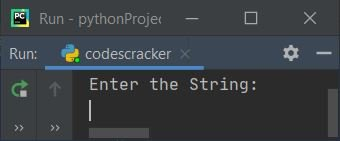Now supply the string say Hello Python, this is codescracker and press `ENTER` key to find and print the largest word from given string as shown in the snapshot given below: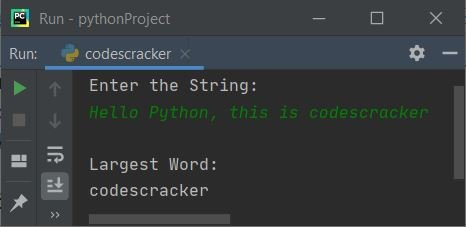#### Modified Version of Previous Program

This is the modified version of previous program. The end used here, to skip insertion of an automatic newline using print().

```print(end="Enter the String: ")
text = input()

text = text.split()
bigWordLen = 0

for wrd in text:
wrdLen = len(wrd)
if wrdLen>bigWordLen:
bigWordLen = wrdLen

print("\nLargest Word(s): ", end="")
for wrd in text:
wrdLen = len(wrd)
if wrdLen==bigWordLen:
print(end=wrd +" ")
print()```

Here is its sample run with user input, Hello there How are You: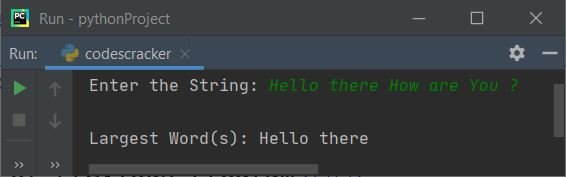## Print Smallest Word in a String

This program find and prints smallest word in a string entered by user. In this program, we've assumed the length of very first word from given string, as the smallest word. Then further compared this length with all the other word's length, and decided which word is the smallest one. Let's have a look at the program and its sample run for clear understanding about it.

```print(end="Enter the String: ")
text = input()

text = text.split()
smallWordLen = len(text)

for wrd in text:
wrdLen = len(wrd)
if wrdLen<smallWordLen:
smallWordLen = wrdLen

print("\nSmallest Word(s): ", end="")
for wrd in text:
wrdLen = len(wrd)
if wrdLen==smallWordLen:
print(end=wrd +" ")
print()```

Here is its sample run with user input, program to find smallest word: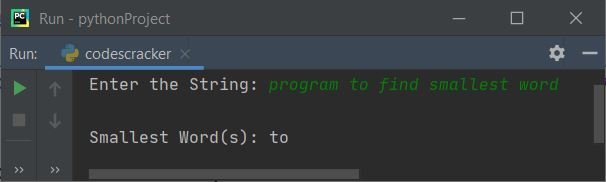## Find Largest and Smallest Word in String

This is the combined version of both the program. That is finding and printing of smallest and largest word in a string.

```print(end="Enter the String: ")
text = input()
text = text.split()
sWrdLen = len(text)
bWrdLen = 0

for wrd in text:
wrdLen = len(wrd)
if wrdLen<sWrdLen:
sWrdLen = wrdLen
if wrdLen>bWrdLen:
bWrdLen = wrdLen

bWrdCount = 0
bWrdList = []
b = 0
sWrdCount = 0
sWrdList = []
s = 0

for wrd in text:
wrdLen = len(wrd)
if wrdLen==bWrdLen:
bWrdCount = bWrdCount+1
bWrdList.insert(b, wrd)
b = b+1
if wrdLen==sWrdLen:
sWrdCount = sWrdCount+1
sWrdList.insert(s, wrd)
s = s+1

if bWrdCount==1:
print("\n---Largest Word---")
print(bWrdList, end="")
else:
print("\n---List of Largest Words---")
for i in range(bWrdCount):
print(bWrdList[i], end=" ")
print()
if sWrdCount==1:
print("\n---Smallest Word---")
print(sWrdList, end="")
else:
print("\n---List of Smallest Words---")
for i in range(sWrdCount):
print(sWrdList[i], end=" ")
print()```

Here is its sample run with user input, Hello student how are you: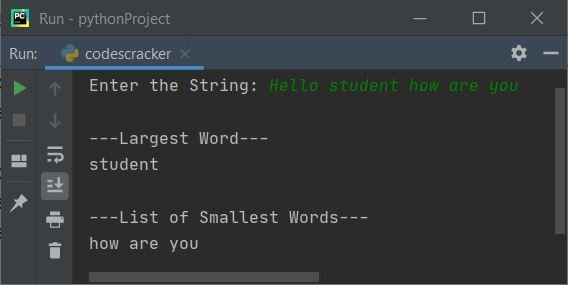### Smallest Program to Find Largest/Smallest Word in String

This is the smallest program that does the same task as previous program does.

```print(end="Enter the String: ")
text = input()
print("\nLargest Word(s): ")
print(*[i for i in text.split() if len(i) == max([len(k) for k in text.split()])])
print("\nSmallest Word(s): ")
print(*[i for i in text.split() if len(i) == min([len(k) for k in text.split()])])```

Here is its sample run with user input, how are you student:Python Online Test

« Previous Program Next Program »